# # 使用Typescript新特性Template Literal Types完善链式key的类型推导

## # 前言

Mpx框架中我们采用了类似 `Vuex` 的数据仓库设计，这使得小程序的数据也可以在框架中得到统一的管理。但由于设计上的原因，这一部分在TS推导的实现上变得异常艰难，其中有一个明显的问题就是链式key的推导：

``````// 一段 mpx-store 代码实例

createStoreWithThis({
// other options ...
actions: {
someAction() {
// 下面这一个dispatch语句如何得到正确的推导？result的类型如何获取？
let result = this.dispatch('deeperActions.anotherAction', 1)
return result
}
},
deps: {
deeperActions: createStoreWithThis({
actions: {
// do something
}
}
}),
// other deps ...
}
})
``````

## # 特性

``````type Color = "red" | "blue";
type Quantity = "one" | "two";

type SeussFish = `\${Quantity | Color} fish`;
//   ^ = type SeussFish = "one fish" | "two fish" | "red fish" | "blue fish"
``````

``````type A = '1' | '2'
type B = 'a' | 'b'
type C = 'C' | 'D'

type D = `\${A}\${B}\${C}`
// type D = "1aC" | "1aD" | "1bC" | "1bD" | "2aC" | "2aD" | "2bC" | "2bD"
``````

## # 可以用来做什么

``````function getValueByPath(object, prop) {
prop = prop || '';
const paths = prop.split('.');
let current = object;
let result = null;
for (let i = 0, j = paths.length; i < j; i++) {
const path = paths[i];
if (!current) break;

if (i === j - 1) {
result = current[path];
break;
}
current = current[path];
}
return result;
}

// 调用例子
getValueByPath({ a: false }, 'a') // false
getValueByPath({ a: { b: { c: 1 } } }, 'a.b') // { c: 1 }
``````

``````type simpleActions = {
}

declare function dispatch<K extends keyof simpleActions>(type: K): ReturnType<simpleActions[K]>

dispatch('actionOne')
// function dispatch<"actionOne">(type: "actionOne"): Promise<number>
``````

``````const actions = {
},
deeperActions: {
anotherAction() {
// do something
return 'result'
},
otherActions: {
}
}
}
}

type Actions = typeof actions

// 编写一个 dispatch 方法，使得：
// let result = dispatch('deeperActions.anotherAction') // 返回类型为： Promise<string>
// let final = dispatch('deeperActions.otherActions.finalAction', 'hello world') // 返回类型为：Promise<boolean>
``````

## # getValueByPath使用TS实现

### # 单层推导

``````declare function getValueByPath(object: Record<any, any>, prop: string): any
``````

``````declare function getValueByPath<T extends Record<any, any>>(object: T, prop: string): any
``````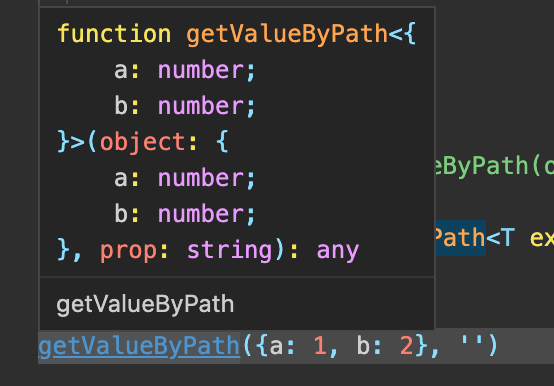``````declare function getValueByPath<T extends Record<any, any>, K extends keyof T>(object: T, prop: K): T[K]

let a = getValueByPath({a: 1, b: '2'}, 'a') // number
let b = getValueByPath({a: 1, b: '2'}, 'b') // string
let c = getValueByPath({a: 1, b: '2'}, 'c') // Argument of type '"c"' is not assignable to parameter of type '"a" | "b"'.ts(2345)
``````

### # 多层推导

``````let a = getValueByPath({ a: { b: { c: 1 } } }, 'a.b')
// Argument of type '"a.b"' is not assignable to parameter of type '"a"'.ts(2345)
``````

``````// 取出对象的所有除了 symbol 以外的key
type StringKeyof<T> = Exclude<keyof T, symbol>

// 将字符串用 . 进行拼接
type CombineStringKey<H extends string | number, L extends string | number> = H extends '' ? `\${L}` : `\${H}.\${L}`
``````

`CombineStringKey` 则是对两个key进行拼接，拼接的结果自然就是我们需要的链式key格式。

``````const symbol1 = Symbol()

type A = {
1: string
a: string
[symbol1]: string
}

type K = StringKeyof<A> // 1 | 'a'

type B = CombineStringKey<'', 'a'>         // "a"
type C = CombineStringKey<B, 'b'>          // "a.b"
type D = CombineStringKey<C, 'c1' | 'c2'>  // "a.b.c1" | "a.b.c2"
type E = CombineStringKey<D, 'd'>          // "a.b.c1.d" | "a.b.c2.d"
``````

``````type deepObj = {
a: number;
b: {
c: string;
d: number;
e: {
f: number;
g: boolean;
};
};
}
``````

``````type ChainKeys<T, P extends string | number = ''> = {
[K in StringKeyof<T>]: T[K] extends Record<any, any> ? ChainKeys<T[K], CombineStringKey<P, K>> : {
[_ in CombineStringKey<P, K>]:T[K]
}
}[StringKeyof<T>]
``````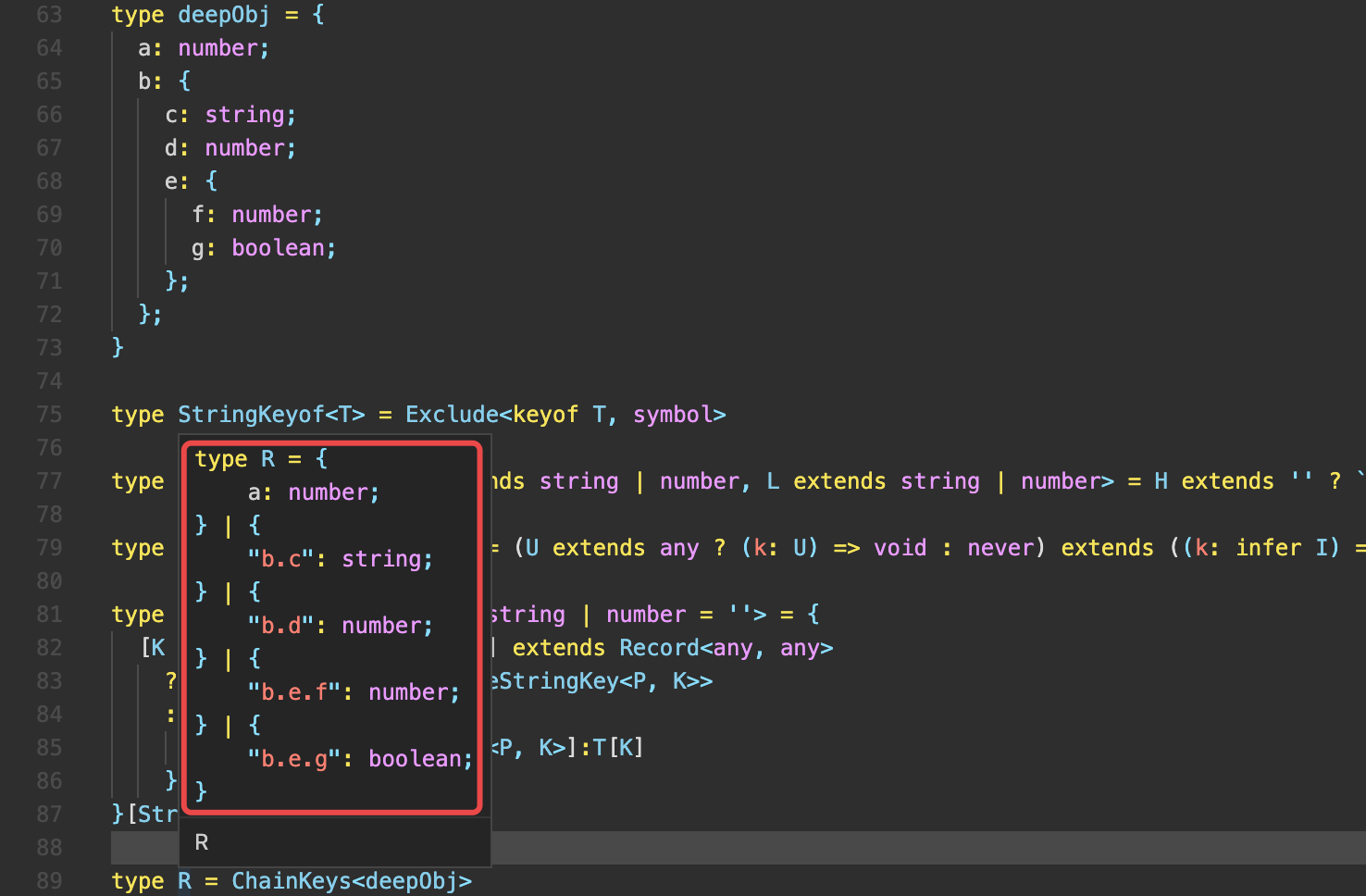``````type ChainKeys<T, P extends string | number = ''> = {
[K in StringKeyof<T>]: T[K] extends Record<any, any>
? Record<CombineStringKey<P, K>, T[K]> & ChainKeys<T[K], CombineStringKey<P, K>>
: {
[_ in CombineStringKey<P, K>]:T[K]
}
}[StringKeyof<T>]
``````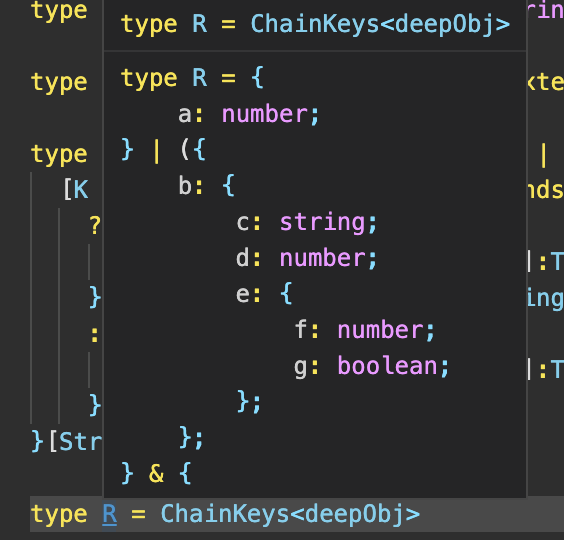``````type T = { a: number } | { b: string }
type K = keyof T // never
// 如何使K的类型为 a ｜ b 呢？
``````

``````type UnionToIntersection<U> = (U extends any ? (k: U) => void : never) extends ((k: infer I) => void) ? I : never;
``````

``````type ChainKeys<T, P extends string | number = ''> = UnionToIntersection<{
[K in StringKeyof<T>]: T[K] extends Record<any, any>
? Record<CombineStringKey<P, K>, T[K]> & ChainKeys<T[K], CombineStringKey<P, K>>
: {
[_ in CombineStringKey<P, K>]:T[K]
}
}[StringKeyof<T>]>
``````

``````type ChainKeys<T, P extends string | number = ''> = UnionToIntersection<{
[K in StringKeyof<T>]: Record<CombineStringKey<P, K>, T[K]> & (T[K] extends Record<any, any> ? ChainKeys<T[K], CombineStringKey<P, K>> : {})
}[StringKeyof<T>]>
``````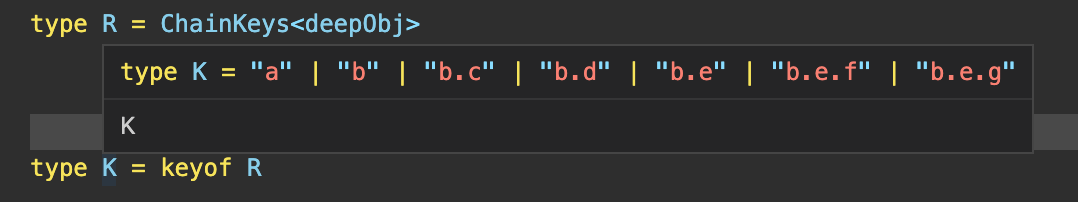``````declare function getValueByPath<T extends Record<any, any>, K extends keyof ChainKeys<T>>(object: T, prop: K): ChainKeys<T>[K]

let a = getValueByPath({ a: { b: { c: 1 } } }, 'a')     // { b: { c: number } }
let b = getValueByPath({ a: { b: { c: 1 } } }, 'a.b')   // { c: number }
let c = getValueByPath({ a: { b: { c: 1 } } }, 'a.b.c') // number
``````

## # 深层次Actions的dispatch函数TS实现

``````interface DeeperActions {
[k: string]: ((...args: any[]) => any) | DeeperActions
}
type ActionChainKeys<T, P extends string | number = ''> = UnionToIntersection<{
[K in StringKeyof<T>]: T[K] extends DeeperActions
? ActionChainKeys<T[K], CombineStringKey<P, K>>
: Record<CombineStringKey<P, K>, T[K]>
}[StringKeyof<T>]>
``````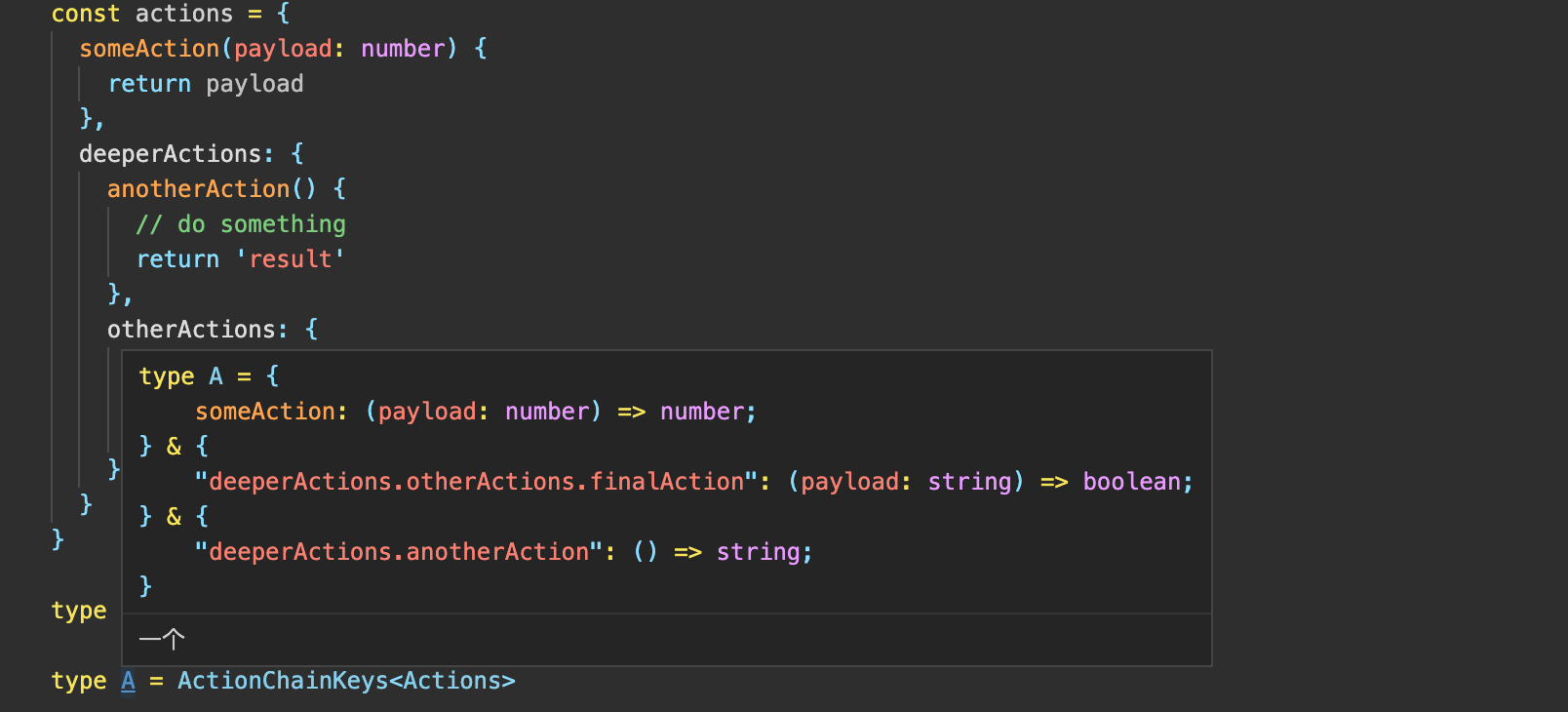`let result = dispatch('deeperActions.anotherAction') // 返回类型为： Promise<string>`

`let final = dispatch('deeperActions.otherActions.finalAction', 'hello world') // 返回类型为：Promise<boolean>`

``````declare function dispatch<K extends keyof ChainingActions>(key: K, ...args: Parameters<ChainingActions[K]>): ReturnType<ChainingActions[K]>
``````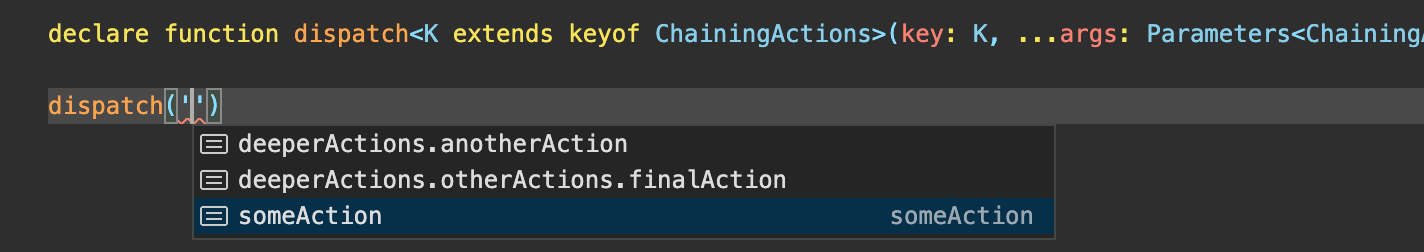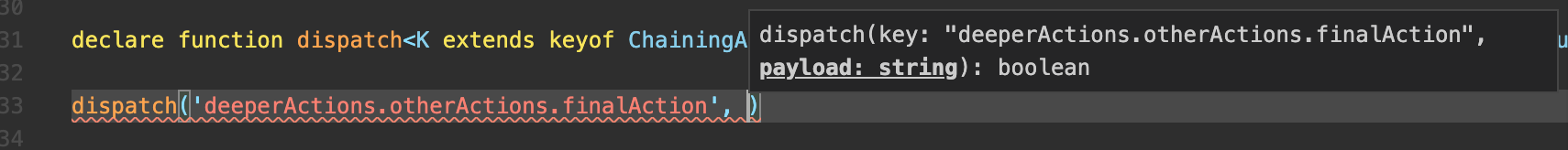``````type Promisify<T> = T extends Promise<any> ? T : Promise<T>

declare function dispatch<K extends keyof ChainingActions>(key: K, ...args: Parameters<ChainingActions[K]>): Promisify<ReturnType<ChainingActions[K]>>

let result = dispatch('deeperActions.anotherAction') // Promise<string>
let final = dispatch('deeperActions.otherActions.finalAction', 'hello world') // Promise<boolean>
``````• 若图片加载失败请访问： http://cdn.qiniu.archerk.com.cn/QQ20210128-162139-HD.gif

## # 结尾

``````type CutChainKeys<T extends string> = T extends `\${infer A}.\${infer B}` ? [A, B] : [T, never]

type GetValueByPath<T extends Record<string, any>, K extends string> = CutChainKeys<K> extends [string, never]
? T[CutChainKeys<K>]
: GetValueByPath<T[CutChainKeys<K>], CutChainKeys<K>>

declare function test<T extends Record<any, any>, K extends string>(o: T, k: K): GetValueByPath<T, K>

let s = test({
a: {
b: 1,
c: {
d: {
e: {
f: 'f'
}
}
}
}
}, 'a.c.d.e.f') // string
``````

## # 题外话：UnionToIntersection

### # Distributive conditional types

Conditional types in which the checked type is a naked type parameter are called distributive conditional types. Distributive conditional types are automatically distributed over union types during instantiation. For example, an instantiation of `T extends U ? X : Y` with the type argument `A | B | C` for `T` is resolved as `(A extends U ? X : Y) | (B extends U ? X : Y) | (C extends U ? X : Y)`.

``````type A = 1 | '2' | 3

type IsNumber<T> = T extends number ? T : never

type B = IsNumber<A> // 1 | 3
``````

### # Type inference in conditional types

Within the `extends` clause of a conditional type, it is now possible to have `infer` declarations that introduce a type variable to be inferred. Such inferred type variables may be referenced in the true branch of the conditional type. It is possible to have multiple `infer` locations for the same type variable.

``````type ReturnType<T> = T extends (...args: any[]) => infer R ? R : any;
``````

``````type Foo<T> = T extends { a: infer U; b: infer U } ? U : never;
type T10 = Foo<{ a: string; b: string }>; // string
type T11 = Foo<{ a: string; b: number }>; // string | number
``````

``````type Bar<T> = T extends { a: (x: infer U) => void; b: (x: infer U) => void }
? U
: never;
type T20 = Bar<{ a: (x: string) => void; b: (x: string) => void }>; // string
type T21 = Bar<{ a: (x: string) => void; b: (x: number) => void }>; // string & number
``````

### # UnionToIntersection 原理探究

``````type UnionToIntersection<U> = (
U extends any
? (k: U) => void
: never
) extends ((k: infer I) => void)
? I
: never;
``````

1、将联合类型的每一项填充至 函数类型 `(k: U) => void` 的参数 `k` 中。

2、使用 `infer` 关键字在逆变位`(k: U) => void` 做推导，将参数的类型推入结果 `I` 中，组成交叉类型。

``````// 联合类型推入函数参数
type UnionToFunction<U> = U extends any ? (k: U) => void : never
// 对函数逆变推导，将参数推导为交叉类型
type FunctionsToIntersection<F> = [F] extends [(k: infer I) => void] ? I : never

type Union = { a: number } | { b: string }

type Fun = UnionToFunction<Union> // ((k: { a: number }) => void) | ((k: { b: string }) => void)

type Intersection = FunctionsToIntersection<Fun> // { a: number } & { b: string }
``````